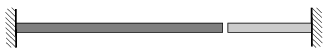Mathematical and Physical Journal
for High Schools
Issued by the MATFUND Foundation
 Already signed up? New to KöMaL?

#Problem P. 4364. (September 2011)

P. 4364. The figure shows two rods, a copper one and an aluminum one, which comes out of a wall. At a temperature of 20 oC the length of the copper rod is 2 m, and the length of the aluminum one is 1 m. The gap between them is 1.3 mm.

a) At what temperature will the gap disappear?

b) What is the size of the gap at a temperature of 0 oC?(4 pont)

Deadline expired on October 10, 2011.

Sorry, the solution is available only in Hungarian. Google translation

Megoldás. $\displaystyle a)$ Kb. $\displaystyle 42^\circ$C-on záródik a rés.

$\displaystyle b)$ Fagyponton kb. 2,5 mm-es a rés.

### Statistics:

 282 students sent a solution. 4 points: 215 students. 3 points: 42 students. 2 points: 17 students. 1 point: 7 students. Unfair, not evaluated: 1 solutions.

Problems in Physics of KöMaL, September 2011